# Time weighted control chart in Excel tutorial

2017-10-20

This tutorial will help you set up and interpret a time weighted control chart in Excel using the XLSTAT statistical software.

## Dataset to generate a time weighted chart

An Excel sheet containing both the data and the results for use in this tutorial can be downloaded by clicking here.

The data are from [Ryan Th. P. (2000), Statistical Methods for Quality Improvement, Second Edition, John Wiley & Sons, New York].

They correspond to simulated data of 20 inspections each having 4 measurements of a production process.

## Time weighted charts

The time weighted charts tool offers you the following chart types:

• CUSUM or CUSUM individual
• UWMA or UWMA individual
• EWMA or EWMA individual

A CUSUM, UWMA or EWMA chart is useful to follow the mean of a production process. Mean shifts are easily visible in the diagrams.

### UWMA and EWMA charts

These charts are not directly based on the raw data. They are based on the smoothed data.

In the case of UWMA charts, the data is smoothed using a uniform weighting in a moving window. Then the chart is analyzed like Shewhart charts.

In the case of EWMA charts, the data is smoothed using an exponential weighting. Then the chart is analyzed like Shewhart charts.

### CUSUM charts

These charts are not directly based on the raw data. They are based on the normalized data.

These charts help to detect mean shifts of a user defined granularity. The granularity is defined by the design parameter k. K is the half of the mean shift to be detected. Normally to detect 1 sigma shifts, k is set to 0.5.

In addition to the control charts connected functions are available:

• Box-Cox transformation
• Process capability
• Tests for normality
• Rules for special causes and Westgard rules
• Run charts

This tutorial explains the ability of the CUSUM charts to detect quicker than a comparable X-bar chart a small mean shift. A process will be simulated that has a mean of 0 and a standard deviation of 1 for the first 12 inspections. Starting with the 13th inspection, the process mean is now 0.5. The standard deviation is unchanged 1. Therefore the first 12 inspections are simulated with a normal law N(0, 1) and the 8 following inspections by the normal law N(0.5, 1). It will be shown using the X-bar chart and the CUSUM chart, how fast this mean shift will be detected.

During this tutorial a one-sided CUSUM chart and a X-bar chart will be used.

## Setting up time weighted charts

Once XLSTAT is activated, select the XLSTAT / SPC / Time weighted command.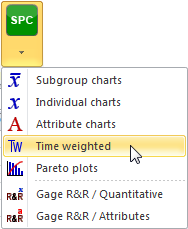When the time weighted dialog box appears, in the Mode tab, chose the CUSUM chart.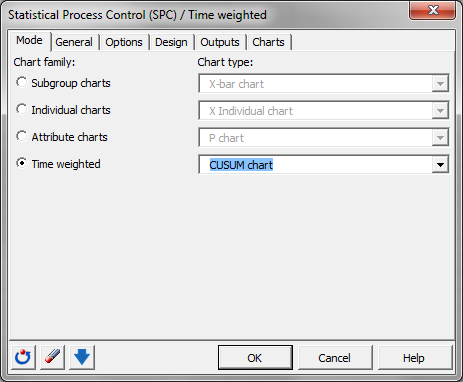Next, move on to the tab General and select the data on the Excel sheet.

Note: There are several ways of selecting data with XLSTAT - for further information, please check the section on selecting data. In this example, the data start from the first row, so it is quicker and easier to use columns selection. This explains why the letters corresponding to the columns are displayed in the selection boxes.

The Data format chosen is Columns because of the format of the input data.

Activate the option Target and enter the mean value of the process 0.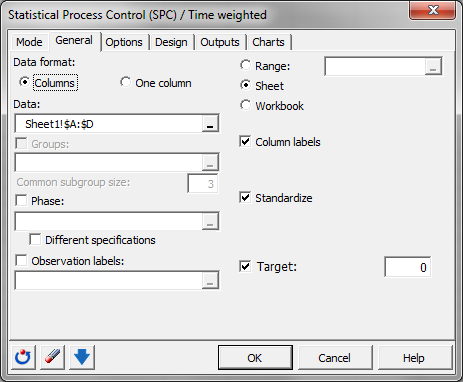In the Options tab, we activate the option Sigma and enter the historical value for sigma: 1.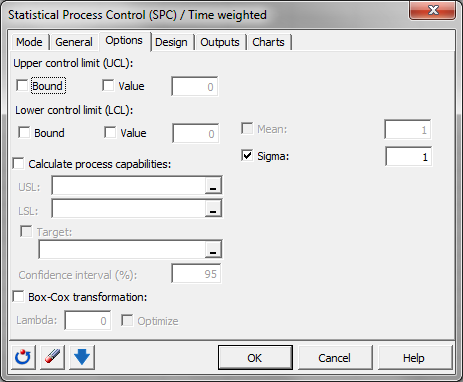In the Design tab, choose the Scheme one sided (LCL/UCL) to obtain the cumulated lower and upper sum.

Enter 4 for h to detect a mean shift, when the cumulated sum has a value of 4 or bigger. Enter 0.5 for k to have a model that detects mean shifts starting at 0.5 sigma or more.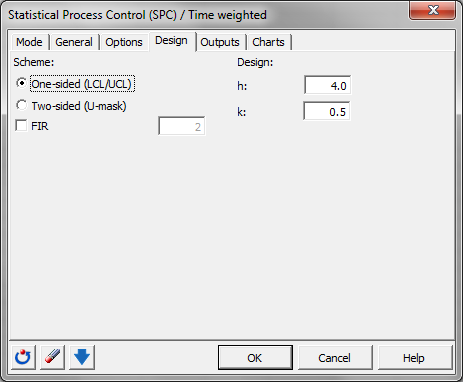In the Outputs tab, we activate all options, and we deactivate all special cause rules.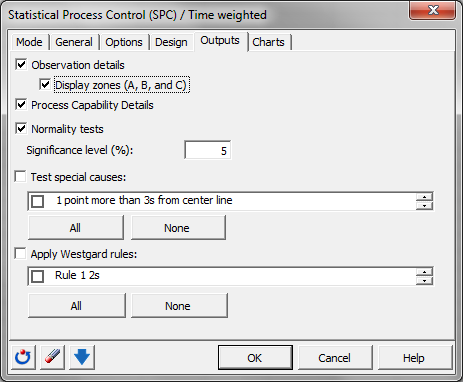In the Charts tab, we activate all options.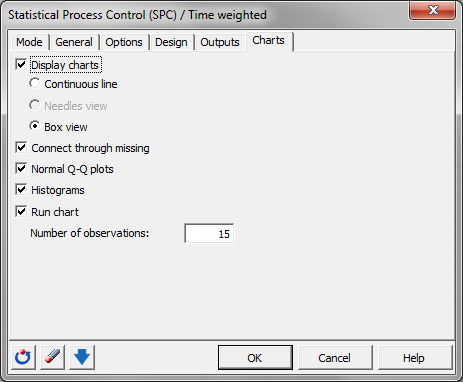The computations begin once you have clicked on OK. You are asked to confirm the number of rows and columns (this message can be bypassed by un-selecting the Ask for selections confirmation in the XLSTAT options panel).

## Interpreting time weighted chart

The first results are the estimated mean and standard deviation values. These values are the same than the historical values entered: 0 and 1.

The following tables with their corresponding chart represent the CUSUM control chart including the different control limits and central lines. In the first table the control limits of the NP control chart are mentioned. They are chosen using the parameter h = 4. LCL equals -4 and UCL equals 4.

Then the data of the CUSUM control chart starting with the group size, the normalized values (z), the lower CUSUM, and the upper CUSUM are displayed. After this the central line (CL), the lower (LCL) and the upper (UCL) control limit and the lower and upper zone limits for the A and B area are displayed for each group.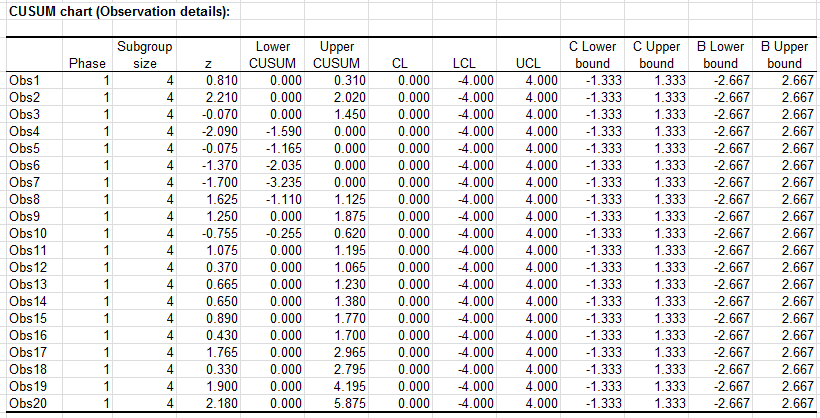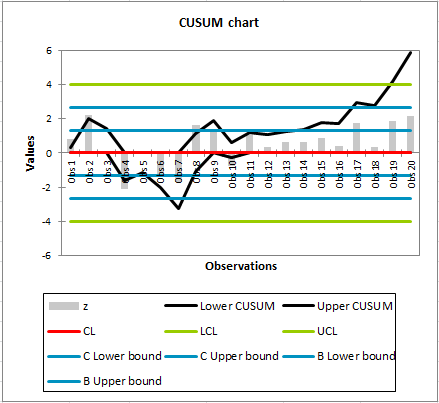We see that the upper CUSUM for inspection 19 has a value of 4.195 and is outside the control limit of 4. This is the detection of the mean shift. It took 7 inspections to detect the shift.

If you draw with the data used in this tutorial a X-bar chart as described in the tutorial on the X-bar chart, even after 20 inspections no value outside the control limits is detected.

The normalized values z in the CUSUM details represent a X-bar chart in a condensed format. If a value is bigger than 3, it is equal to a value in the X-bar chart bigger than 3 sigma, the usual upper control limit for a X-bar chart. In this case here all normalized values z are smaller than 3.

During this tutorial we could see that the CUSUM chart is more efficient to detect small mean shifts than an X-bar chart.

#### Contact our technical support team: support@xlstat.com

https://cdn.desk.com/
false
desk